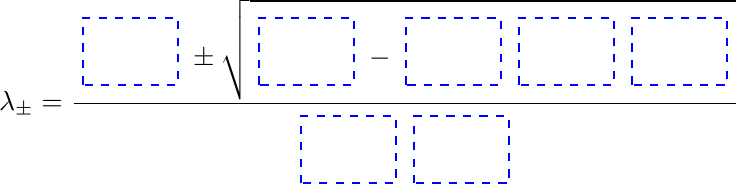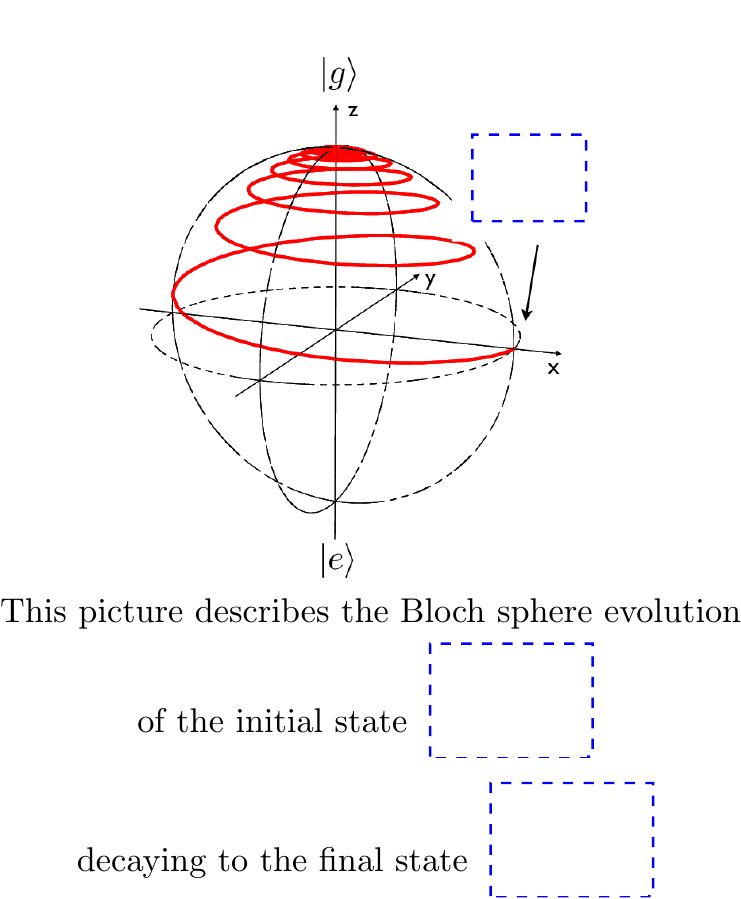Generate edX drag-and-drop problems using compilation from latex

# latex2dnd

Generate an edX drag-and-drop problem, complete with draggable labels, problem image, and solution image, from an input latex file.## Installation

pip install -e git+https://github.com/mitocw/latex2dnd.git#egg=latex2dnd


Note that pdftoppm is needed. With osX and macbrew:

brew install netpbm poppler


Also imagemagick is required

brew install imagemagick


A working latex installation is also required.

## Usage

Usage: latex2dnd [options] [filename.tex | filename.dndspec]

Options:
--version             show program's version number and exit
-h, --help            show this help message and exit
-v, --verbose         verbose messages
-V, --very-verbose    very verbose messages
-C, --can-reuse-labels
allow draggable labels to be reusable
-s, --skip-latex-compilation
skip latex compilation
-d OUTPUT_DIR, --output-directory=OUTPUT_DIR
Directory name for output PNG files
-c CONFIG_FILE, --config-file=CONFIG_FILE
-u IMAGE_URL, --url-for-images=IMAGE_URL
base URL for images
-r RESOLUTION, --resolution=RESOLUTION
Resolution of PNG files in DPI (default 300), can set to 'max' to auto-scale
--cfn=CUSTOM_CFN      Name of python script check function to use for drag-drop checking
--output-tex          Final output should be a tex file (works when input is a *.dndspec file)
--output-catsoop      Final output should be a markdown file for catsoop
--cleanup             Remove old solution image files, and tmp.pdf
--nonrandom           Do not use a random string in the solution filename
--tex-options-override
allow options in tex or dndspec file to override command line options


## Example

See *.tex and *.dndspec files in the examples directory.Or: get quadratic.tex from the latex2dnd/testtex directory, and run this:

this will generate 220dpi images, with the files:

quadratic_dnd.xml -- edX drag-and-drop question XML
./quadratic_dnd_sol.png -- dnd problem solution image
9 dnd draggable image labels:


Insert this into your course with (if you're using https://github.com/mitocw/latex2edx):

\begin{edXproblem}{Latex to drag and drop test}{}

\end{edXproblem}


and copy the png files to /static/images/quadratic/

## LaTeX Macros

latex2dnd works by introducing three important new macros:

1. \DDlabel{label_name}{label_contents}
-- label_name = alphanumeric name (no spaces) for the draggable label -- label_contents = latex string for contents of label (math ok, e.g. $\sqrt{a+b}$)

2. \DDBox{box_name}{width}{height}{label_name} -- box_name = alphanumeric name (no spaces) for the target box -- width, height = size of target box -- label_name = name of label which gives correct label to go in this box (the answer)

3. \writeDDlabels[height] -- height = optional argument which specifies the height of the labels (widths are automatic)

Here is an example, giving the LaTeX code for a drag-and-drop question asking for the quadratic equation roots formula to be filled in:

\documentclass{article}
\input{latex2dnd}

\begin{document}

% define drag-drop labels
\DDlabel{term1}{$-b$}
\DDlabel{term1p}{$+b$}
\DDlabel{term2}{$b^2$}
\DDlabel{dubexp}{$b^{2^\alpha}$}
\DDlabel{dubsub}{$b_{2_\alpha}$}
\DDlabel{fac}{$-4ac$}
\DDlabel{facp}{$+4ac$}
\DDlabel{ta}{$+2a$}
\DDlabel{tam}{$-2a$}

% shorthand macro to make all boxes the same size (6 by 4)
\newcommand\DDB{\DDbox{#1}{6ex}{4ex}{#2}}

% the formula with boxes
$$\lambda = \frac{\DDB{1}{term1}\pm \sqrt{\DDB{2}{term2}\DDB{3}{fac}}}{\DDB{4}{ta}}$$

% output labels, with fixed box heights
\writeDDlabels[4.3ex]

\end{document}


Note that more labels are defined than just the correct ones. This is the XML generated from the above example:

    <span>
<customresponse>
<target id="1" x="70" y="19" w="123" h="74"/>
<target id="2" x="283" y="19" w="123" h="74"/>
<target id="3" x="427" y="19" w="122" h="74"/>
<target id="4" x="249" y="129" w="122" h="74"/>
</drag_and_drop_input>
caset = [{'term1': '1'}, {'term2': '2'}, {'fac': '3'}, {'ta': '4'}]
ca = [ {"draggables": ca.keys(),"targets": ca.values(),"rule":"exact"} for ca in caset ]
correct = ["correct"]
else:
correct = ["incorrect"]
</customresponse>
<solution>
</solution>
</span>


## Simplified DND Specification

LaTeX is powerful, but can be fragile. A simpler way to write DND problems is to use a "dndspec" specification file; this provides a simple plain-text based representation of a DND problem.

Example:

MATCH_LABELS: G,m_1,m_2,R
BEGIN_EXPRESSION
\bea
\frac{ G m_1 m_2 }{ R }
\nonumber
\eea
END_EXPRESSION
CHECK_FORMULA: G * m_1 * m_2 / R


This input.dndspec file describes a DND problem with the labels

\DDlabel[G]{G}{$G$}
\DDlabel[m1]{mone}{$m_1$}
\DDlabel[m2]{mtwo}{$m_2$}
\DDlabel[R]{R}{$R$}


the DND expression (with boxes):

\bea
\frac{ \DDB{1}{G} \DDB{2}{mone} \DDB{3}{mtwo} }{ \DDB{4}{R} }
\nonumber
\eea


where this tex macro is automatically defined for the box:

\newcommand\DDB{\DDbox{#1}{8ex}{4ex}{#2}}


and this check formula:

\DDformula{  () * () * () / ()  }{ G,m1,m2,R@1,1,1,1:20,20,20,20\#20 }{  G * m1 * m2 / R  }{}


Compile this using

latex2dnd -v input.dndspec


and you'll get input.tex as the DND latex file (which can be edited for futher customizations), together with all the usual output of latex2dnd.

More formally:

DELIMETER: <character to use as a delimeter: defaults to ,>
MATCH_LABELS: <comma separated list of labels appearing in EXPRESSION which should be made into boxes>
DISTRACTOR_LABELS: <comma separated list of labels to be shown as draggables>
ALL_LABELS: <comma separated list of MATCH and DISTRACTOR labels, in desired order, to be shown as draggables>
MATH_EXP: <label>, <math_exp_for_label>  (may be used multiple times; each use should be for a single label)
BEGIN_EXPRESSION
<latex expression containing MATCH labels, with spaces around every label>
END_EXPRESSION
CHECK_FORMULA: <text representation of correct formula, using math expression version of the MATCH labels, to be used for checking>
CHECK_FORMULA_BOXES: <formula using [#], where [#] is the MATCH label number; needed if MATCH labels appear in more than one input box>
TEST_CORRECT: formula which should evaluate to being correct
TEST_INCORRECT: formula which should evaluate to being incorrect
BOX_HEIGHT: draggable label box height to use
BOX_WIDTH: draggable label box width to use
EXTRA_HEADER_TEX: a line with extra latex commands to be inserted into the header (may be used multiple times)
OPTIONS: <dnd_options string>


There should be no leading spaces / indentation on lines with keywords (like MATCH_LABELS).

Here are some additional useful macros:

\DDoptions{} -- extra configuration parameters, e.g. HIDE_FORMULA_INPUT, CAN_REUSE, and ALLOW_EMPTY

\DDformula{ pattern }{ samples }{ expected } -- use formula checking to test correctness of drag-and-drop response. For example:

\DDformula{  *  *  /  }{ G,m_1,m_2,d@1,1,1,1:20,20,20,20\#40 }{G*m_1*m_2/d^2}{}


\DDtest{ correct | incorrect }{ answer_box_ids }{ draggable_ids } -- unit test to perform on DDformula expressions, e.g.

\DDtest{correct}{1,2,3,4}{G,m2,m1,d2}
\DDtest{incorrect}{1,2,3,4}{G,d2,m1,m2}


## Notes

latex2dnd uses the "-region" feature of imagemagick's convert command, to white out solution boxes and generate the problem image. Version 7.0.4 of imagemagick appears to have a bug in how it handles "-region", causing the region specification to be ignored, and thus producing blank problem images (everything whited out). If you encounter this problem, one workaround is to back out to version 6.9.1 of imagemagick. For example, under OSX with homebrew, do:

brew switch imagemagick 6.9.1-10


## History

* v0.9.0: python package, with unit tests
*     .1: add -C (can_reuse) flag
*     .2: improved box alignment and pixel position computation
*     .3: center labels in boxes; custom code when can_reuse=true
*     .5: add \DDoptions{HIDE_FORMULA_INPUT} handling to not display formula input
*     .6: add \DDoptins{CUSTOM_CFN=xxx} and --cfn=xxx command line for custom DND result check function
* v1.0.0: add unit tests for DDformula, which automatically verify expected response is checked properly
* v1.1.0: implement dndspec, a simplified DND problem specification language
*     .1: more improvements to dndspec; use random string in solution image filename; add examples


## Project details

This version1.2.01.1.11.1.01.0.00.9.60.9.40.9.30.9.20.9.10.0.9

Uploaded source